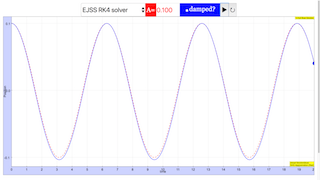intro

Harmonic and Anharmonic Oscillations of a Boat

Developed by E. Ayars

Easy JavaScript Simulation by Fremont Teng and Loo Kang Wee

Simple Harmonic Oscillation (SHO) is everyone’s favorite type of periodic motion. It’s simple, easy to understand, and has a known solution. But SHO is not the only kind of periodic motion. In this set of exercises we’ll investigate an anharmonic oscillator. We’ll explore characteristics of its motion, and try to get an understanding of the circumstances under which the motion can be approximated as simple harmonic.

Subject Area Mechanics First Year and Beyond the First Year Python and Mathematica Easy JavaScript Simulation Students who complete these exercises will be able to use an ODE solver to develop a qualitative understanding of the behavior of an anharmonic oscillator. Most students gain a good understanding of the behavior of harmonic oscillators in their introductory classes, but not all oscillators are harmonic. Here we investigate an idealized boat hull which exhibits asymmetric oscillation: the restoring force is stronger for displacements in one direction than in the other. This results, for large oscillations, in a visibly non-sinusoidal oscillation. For small oscillations, the motion is approximately simple harmonic again. 120 min

instructorguide

This set of exercises guides students through the development of an anharmonic oscillator, then lets them use an ODE solver to plot the motion. It does not describe how to use an ODE solver. Emphasis is on qualitative understanding of the system, although if the instructor chooses it is certainly possible to use real parameters (${\rho }_{water}$, dimensions of boat, etc) to obtain an “engineer’s answer”.

The problem is accessible to first-year students, but it may also be of interest to students in higher-level courses.

There is opportunity to discuss limitations of the model on the final problem. In this problem, if the amplitude is high enough that the boat gets out of the water, the model in the ODE breaks down in interesting and spectacular ways.

The pseudocode, Mathematica notebook, python code, and sample solutions of this Exercise Set assume the use of built-in differential equation solving capabilities. Depending on instructor preference, it may be desirable for students to have experienced the direct application of a finite-difference algorithm to a variety of first-order differential equations before using a computational ‘black box.’

theory

The simplest example of Simple Harmonic Oscillation (SHO) is a mass hanging on a spring. In equilibrium, the string will have stretched some equilibrium distance ${x}_{o}$, so that the weight of the mass $mg$ is balanced by the spring force $-k{x}_{o}$. Any additional motion $x$ of the spring will result in an unbalanced force:

$\begin{array}{}\text{(1)}& \mathrm{\Sigma }F=ma=mg-k\left({x}_{o}+x\right)\end{array}$
$\begin{array}{}\text{(2)}& m\frac{{d}^{2}x}{d{t}^{2}}=\left(mg-k{x}_{o}\right)-kx\end{array}$
$\begin{array}{}\text{(3)}& \frac{{d}^{2}x}{d{t}^{2}}=-\frac{k}{m}x\end{array}$

This last equation is in the form of the equation for SHO:

$\begin{array}{}\text{(4)}& \frac{{d}^{2}x}{d{t}^{2}}=-{\omega }^{2}x\end{array}$

where $\omega \equiv \sqrt{\frac{k}{m}}$ in this case. Knowing $\omega$, we can then go on to do whatever we need to do with the problem. The period of the motion is $T=\frac{2\pi }{\omega }$, the position as a function of time will be $x\left(t\right)=A\mathrm{cos}\left(\omega t+\varphi \right)$, etc.

We can use this “known solution” to SHO any time we can get the equation of motion into the form of the SHO differential equation. If the second derivative of a variable is equal to a negative constant times the variable, the solution is SHO with $\omega$ equal to the square root of that constant.

Even when we can’t get the equation of motion for a system into the form of SHO, we can often approximate the motion as SHO for small oscillations. The classic example of this is the simple pendulum. The equation of motion for the pendulum is

$\begin{array}{}\text{(5)}& \frac{{d}^{2}\theta }{d{t}^{2}}=-\frac{g}{L}\mathrm{sin}\theta \end{array}$

This equation is not SHO, but for small $\theta$, $\mathrm{sin}\theta \approx \theta$ so we can approximate the motion as SHO as long as the oscillations are small.

In the case of our wedge-shaped boat, the equation of motion is

$\begin{array}{}\text{(6)}& \frac{{d}^{2}\gamma }{d{t}^{2}}=-{\omega }_{o}^{2}\left(1+\frac{\gamma }{2}\right)\gamma \end{array}$

This is not SHO! If $\gamma$ is small, it is approximately SHO, but only approximately.

Note: The Mathematica and Python solutions here make use of built-in tools for solving differential equations. For other implementations the reader is directed to Numerical Recipes in C, or equivalent text, which will provide an introduction and theoretical description of the numerical approach to solutions via finite-difference methods.

exercises

## Exercise 1: A straight-sided boat

Imagine a boat with vertical sides, floating. There will be two forces on this boat: its weight $mg$, and the buoyant force $-\rho gV$. (I’ve defined down to be the positive direction, here, so the buoyant force is negative since it’s upwards.) The volume $V$ in the buoyant force component is the volume of water displaced by the boat: in other words, the volume of boat that is below water level. In equilibrium, the bottom of the boat will be some distance ${x}_{o}$ below the water, so $V=A{x}_{o}$, where $A$ is the area of the boat at the waterline.

If we then push the boat down some additional distance $x$ and let go, the boat will bob up and down.

1. Show that the boat’s vertical motion is SHO. It will probably be helpful to follow the same pattern as for the introduction example: the key is to get the equation in the form of the equation for SHO.
2. What is the period of the boat’s motion?

## Exercise 2: A V-hulled boat

Instead of a straight-sided boat, imagine a boat with a V-shaped hull profile, as shown below.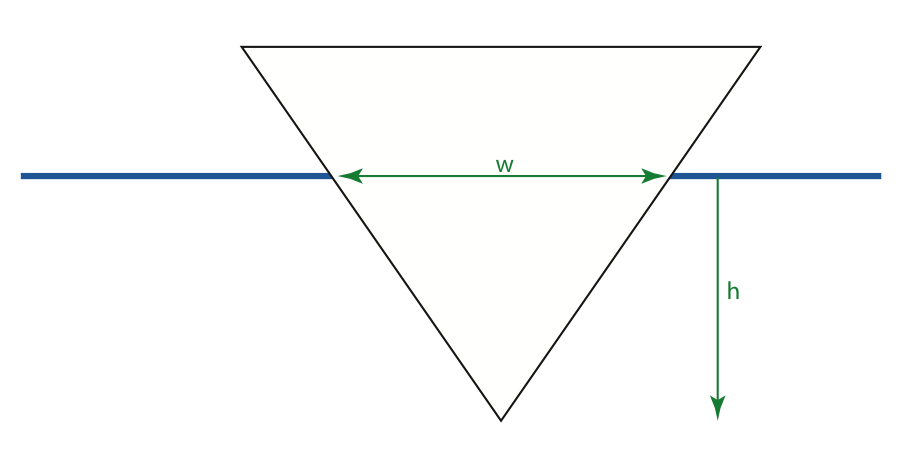The width $w$ of the hull at the waterline depends on the depth below waterline $h$:

$\begin{array}{}\text{(7)}& w=\beta h\end{array}$

The volume of water displaced by this boat is the area of the triangle below water level, times the length of the boat:

$\begin{array}{}\text{(8)}& V=\frac{1}{2}\beta {h}^{2}L\end{array}$
1. Show that the vertical motion of this boat is not SHO. Replace $h$ with $\left({y}_{o}+y\right)$, where ${y}_{o}$ is the equilibrium depth, and follow the example in the introduction.
2. Rearrange the equation you got in the previous problem to be as close to SHO as possible by putting it in this form:

$\begin{array}{}\text{(9)}& \frac{{d}^{2}y}{d{t}^{2}}=-{\omega }_{o}^{2}\left(1+\frac{y}{2{y}_{o}}\right)y\end{array}$

In this form, you can see that if $\frac{y}{2{y}_{o}}$ is small, the motion is approximately SHO with angular frequency ${\omega }_{o}$. What is that ${\omega }_{o}$? What must be small for this approximation to be valid?

3. If $\frac{y}{2{y}_{o}}$ is not small, would the period of the boat’s oscillation be larger or smaller than ${T}_{o}=\frac{2\pi }{{\omega }_{o}}$? Use a numeric solution of the equation of motion for the boat to verify your answer.

4. Plot the motion of the boat for various amplitudes. In addition to effects on $T$, how else does the motion differ from SHO? It will be helpful to plot both the solution to the differential equation and the SHO approximation,

$\begin{array}{}\text{(10)}& y\left(t\right)={y}_{max}\mathrm{cos}\left({\omega }_{o}t\right)\end{array}$

5. We’ve neglected viscous damping, which is a bad idea in liquids! Redo your calculations, and plots, adding a viscous damping force

$\begin{array}{}\text{(11)}& {F}_{v}=-\delta \frac{dy}{dt}\end{array}$

vhull.py

#!/usr/bin/env python

'''

vhull.py

Eric Ayars

June 2016

A solution to the vertical motion of a V-hulled boat.

'''

from pylab import *

from scipy.integrate import odeint

# definitions

w = 1.0 # Well, we need to pick something. Might as well

# normalize the frequency. :-)

delta = 0.2 # not sure what this should be, play with it!

def vhull_ODE(s,t):

# derivs function for v-hull ODE, without viscous damping

g0 = s

g1 = -w*(1+s/2)*s

return array([g0,g1])

def vhull_ODE2(s,t):

# derivs function for v-hull ODE, with viscous damping added

g0 = s

g1 = -w*(1+s)*s - delta*s

return array([g0,g1])

# Now set up the solutions.

time = linspace(0,20,500) # 500 points on interval [0,20]

# And now plot some things!

# Here's a low-amplitude one, should be approximately SHO.

A = 0.1 # Fairly small amplitude

yi = array([A,0.0])

y = answer[:,0] # position is just the first row

plot(time,y, 'b-', label="Actual solution")

plot(time,A*cos(w*time), 'r-', label="SHO approximation")

A = 0.5 # Not small amplitude

yi = array([A,0.0])

y = answer[:,0] # position is just the first row

plot(time,y, 'b-')

plot(time,A*cos(w*time), 'r-')

'''

# For damped motion, just use vhull_ODE2() instead of vhull_ODE.

A = 0.5

yi = array([A,0.0])

y = answer[:,0] # position is just the first row

plot(time,y, 'b-', label="Actual solution")

plot(time,A*cos(w*time), 'r-', label="SHO approximation")

'''

title("V-Hull Boat Solution")

xlabel("time")

ylabel("Position")

legend(loc="lower right")

show()

solutions

## Exercise 1: A straight-sided boat

For a boat with vertical sides, the equation of motion can be derived by starting with Newton’s second law:

$\begin{array}{}\text{(12)}& \mathrm{\Sigma }F=mg-\rho gA\left({y}_{o}+y\right)=m{a}_{y}\end{array}$

where $A$ is the area of the boat’s cross-section at the waterline. ${y}_{o}$ is the equilibrium depth, so $mg=\rho gA{y}_{o}$and

$\begin{array}{}\text{(13)}& {a}_{y}=\frac{{d}^{2}y}{d{t}^{2}}=-\frac{\rho gA}{m}y\end{array}$

This is in the form of the equation for SHO, with

$\begin{array}{}\text{(14)}& \omega =\sqrt{\frac{\rho gA}{m}}\end{array}$

The period of the boat’s oscillation will be

$\begin{array}{}\text{(15)}& T=2\pi \sqrt{\frac{m}{\rho gA}}\end{array}$

## Exercise 2: A V-hulled boat

The derivation for the V-hulled boat follows the same general procedure.

$\begin{array}{}\text{(16)}& V=\frac{1}{2}\beta {h}^{2}L=\frac{1}{2}\beta L\left({y}_{o}+y{\right)}^{2}\end{array}$
$\begin{array}{}\text{(17)}& \mathrm{\Sigma }F=m\frac{{d}^{2}y}{d{t}^{2}}=mg-\frac{1}{2}\rho g\beta L\left({y}_{o}^{2}+2{y}_{o}y+{y}^{2}\right)\end{array}$

The equilibrium position is given by ${y}_{o}$, so $mg-\frac{1}{2}\rho g\beta L{y}_{o}^{2}=0$ and

$\begin{array}{}\text{(18)}& \frac{{d}^{2}y}{d{t}^{2}}=-\frac{\rho g\beta L}{2m}\left(2{y}_{o}y+{y}^{2}\right)\end{array}$

This can be further simplified as

$\begin{array}{}\text{(19)}& \frac{{d}^{2}y}{d{t}^{2}}=-\frac{\rho g\beta L{y}_{o}}{m}\left(1+\frac{y}{2{y}_{o}}\right)y\end{array}$

From that equation we can see that if $\frac{y}{2{y}_{o}}$ is small, then the motion is approximately SHO with

$\begin{array}{}\text{(20)}& {\omega }_{o}=\sqrt{\frac{\rho g\beta L{y}_{o}}{m}}\end{array}$

There are an awful lot of constants in that equation, so to simplify our numeric calculations let’s just redefine things.

$\begin{array}{}\text{(21)}& \frac{{d}^{2}y}{d{t}^{2}}=-{\omega }_{o}^{2}\left(1+\frac{y}{2{y}_{o}}\right)y\end{array}$

We can also define the unitless variable $\gamma \equiv \frac{y}{{y}_{o}}$, which allows us to rewrite the equation in (almost) unit-independent form:

$\begin{array}{}\text{(22)}& \frac{{d}^{2}\gamma }{d{t}^{2}}=-{\omega }_{o}^{2}\left(1+\frac{\gamma }{2}\right)\gamma \end{array}$

That’s the equation we need to send through the ODE solver.

The equation is approximately SHO if the amplitude is small. The easist way to control the amplitude is to set initial position to ${y}_{i}$, with ${v}_{i}=0$, so that the amplitude is ${y}_{i}$.

Setting different values of amplitude gives different behavior: for small amplitude (${\gamma }_{o}=0.1$) the result is nearly indistinguishable from SHO, but for larger values both the period and symmetry change. The figure below shows that at ${\gamma }_{o}=0.5$, the boat spends more time higher (the graph is inverted, since down is positive in our initial setup) and the period lengthens relative to the SHO approximation.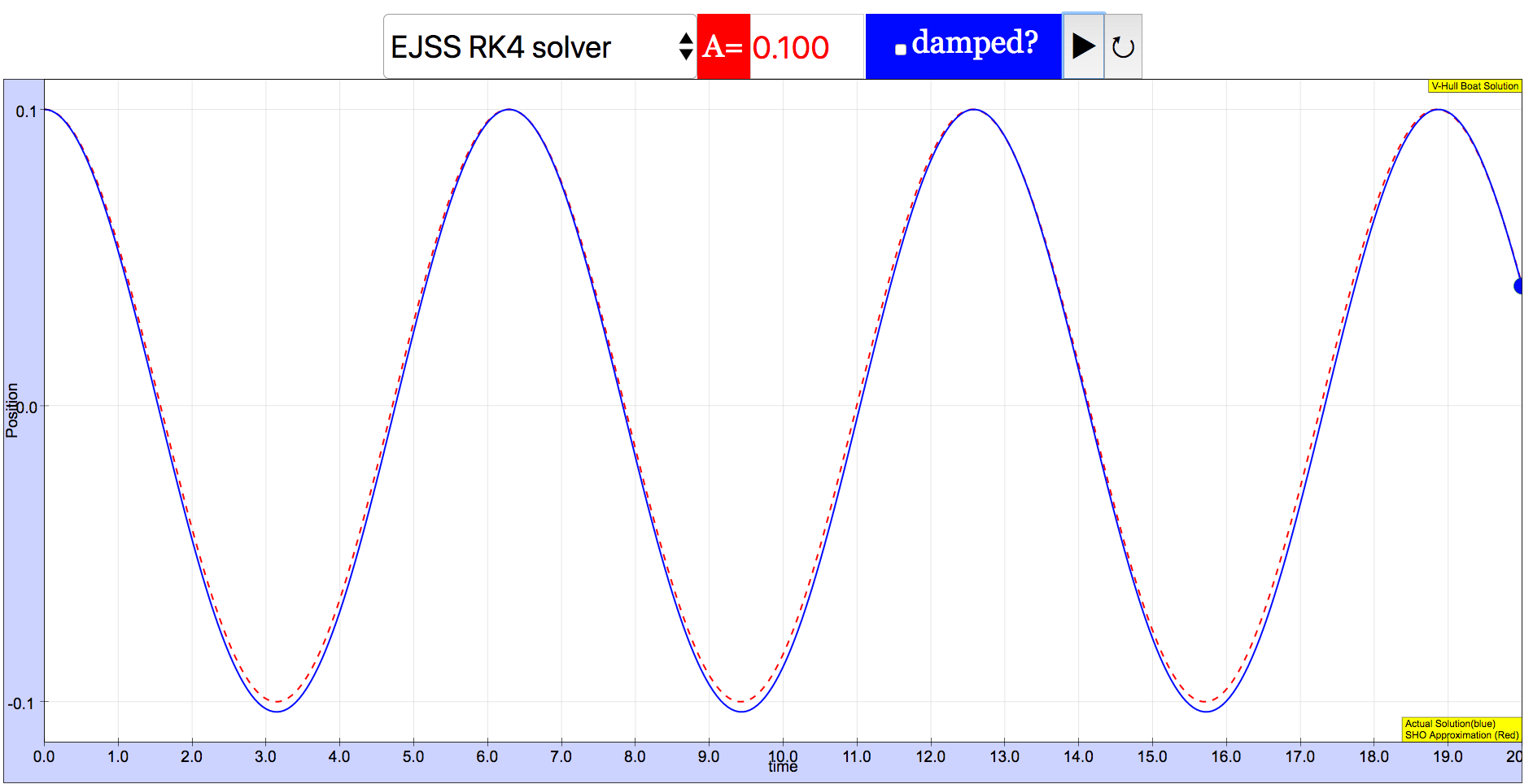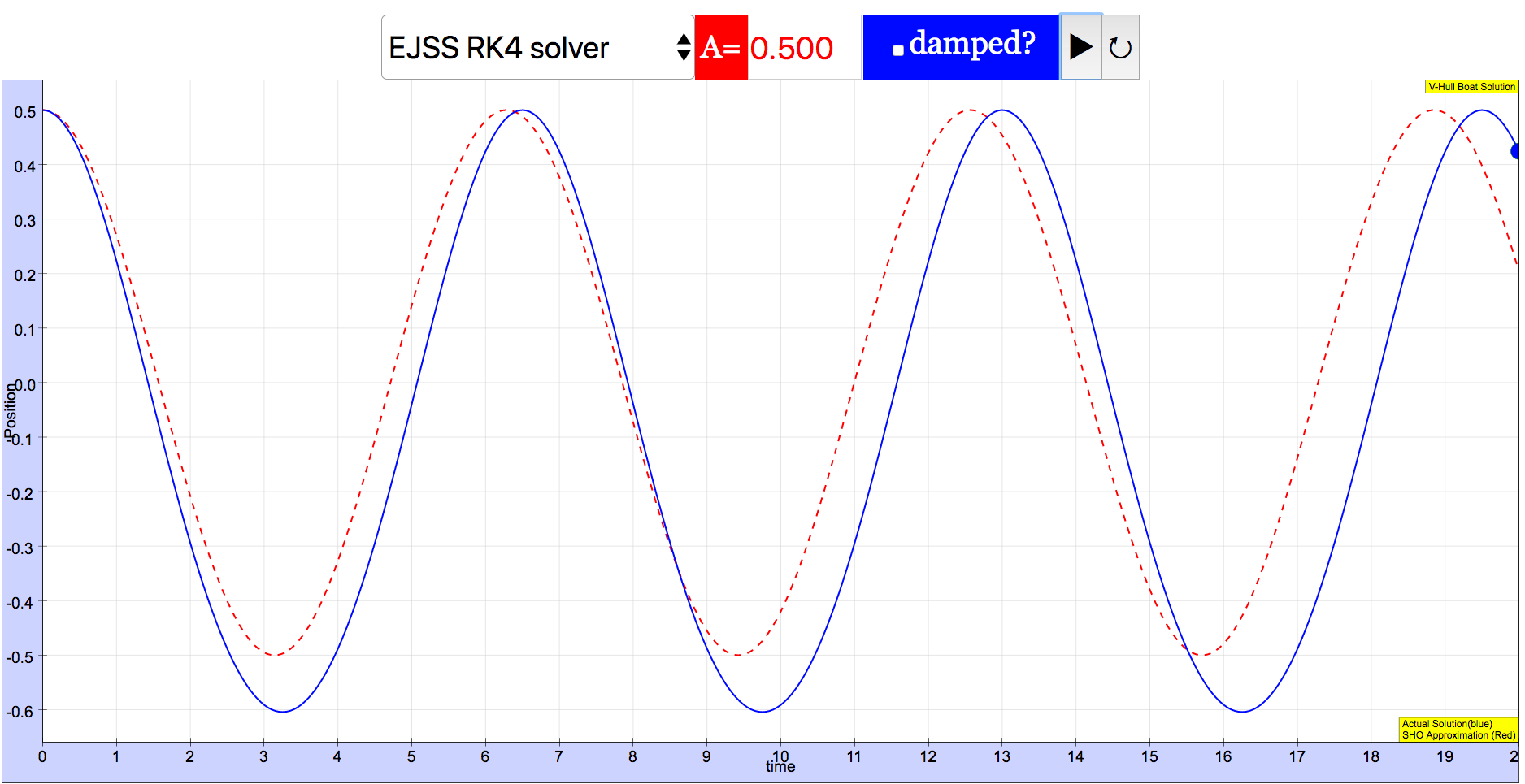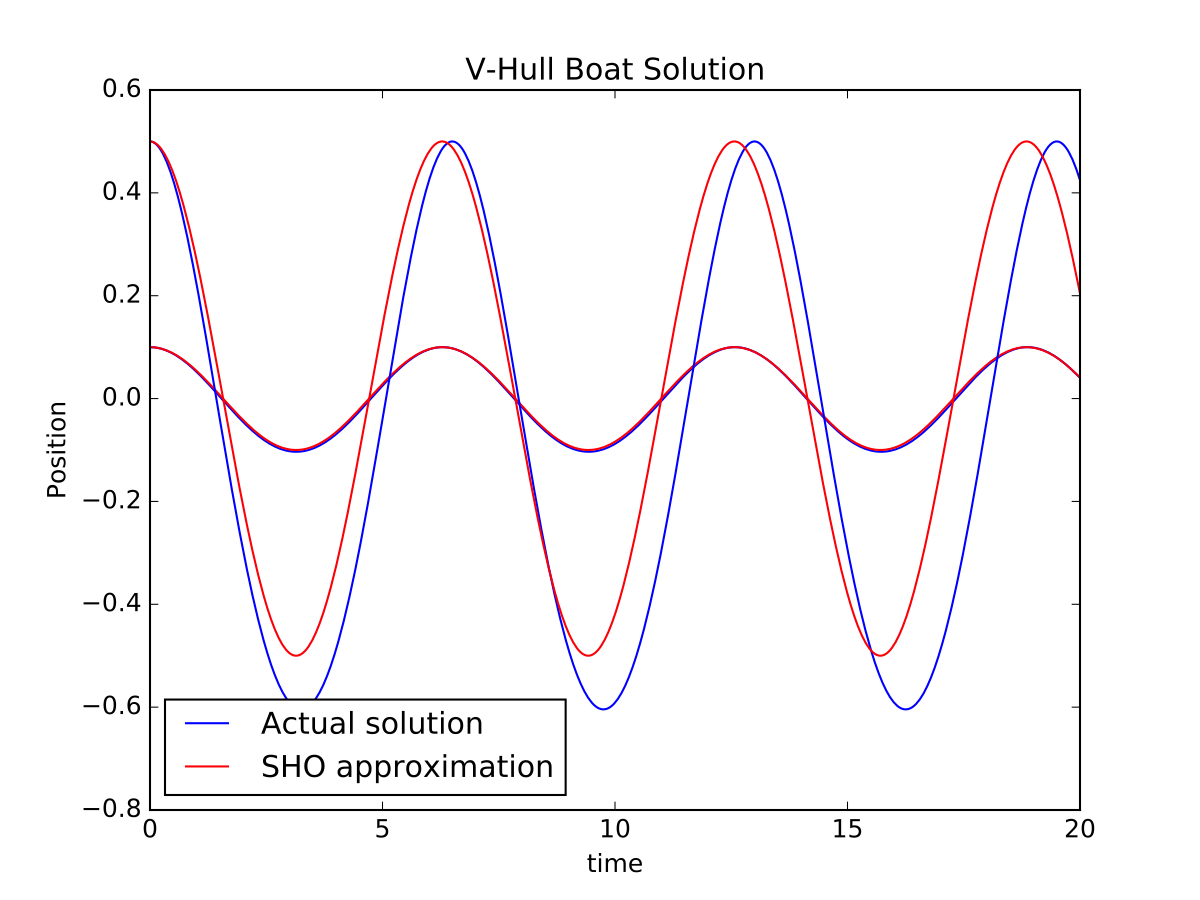These effects are even more prominent at larger values of ${\gamma }_{o}$, as shown in the next figure.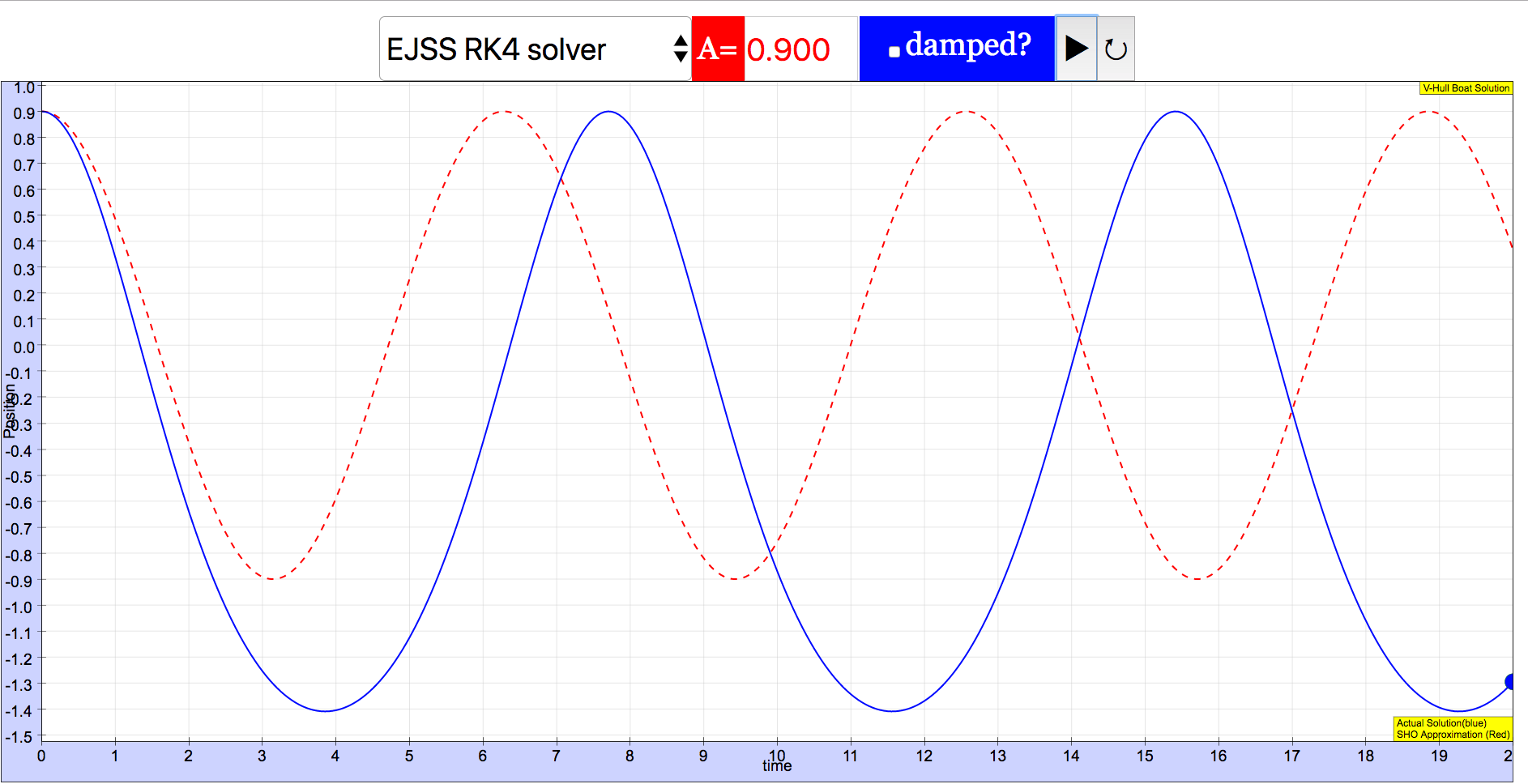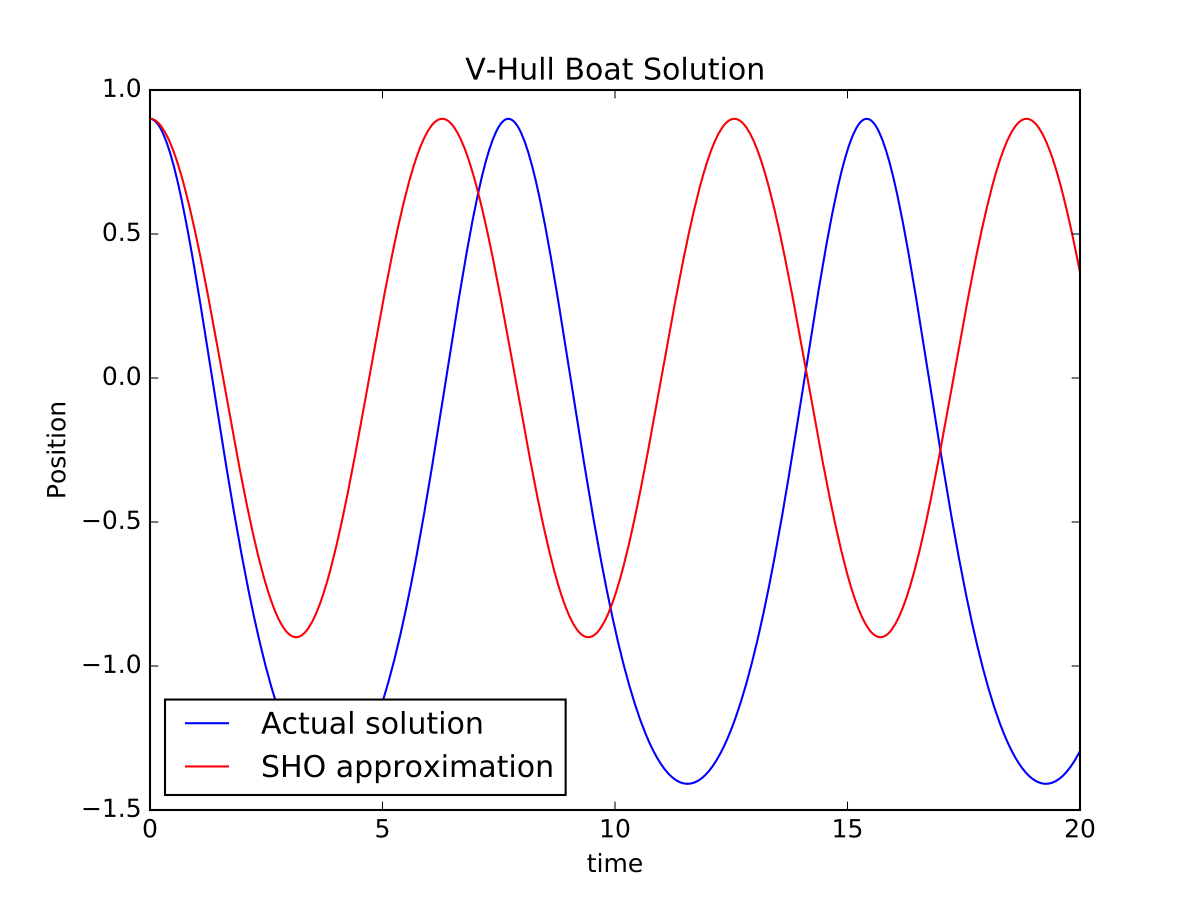There’s an opportunity here for the model to break down. If the boat gets completely out of the water, then the width of the boat becomes negative (ok, it doesn’t really but it does in the model) so then the volume becomes negative and the buoyant force becomes negative and the boat leaps away from the water exactly the way that real boats don’t. Be prepared to discuss this interesting result should the students get the amplitude too high.

Adding damping is relatively easy: just add another force term in the definition of the ODE. Results are shown below.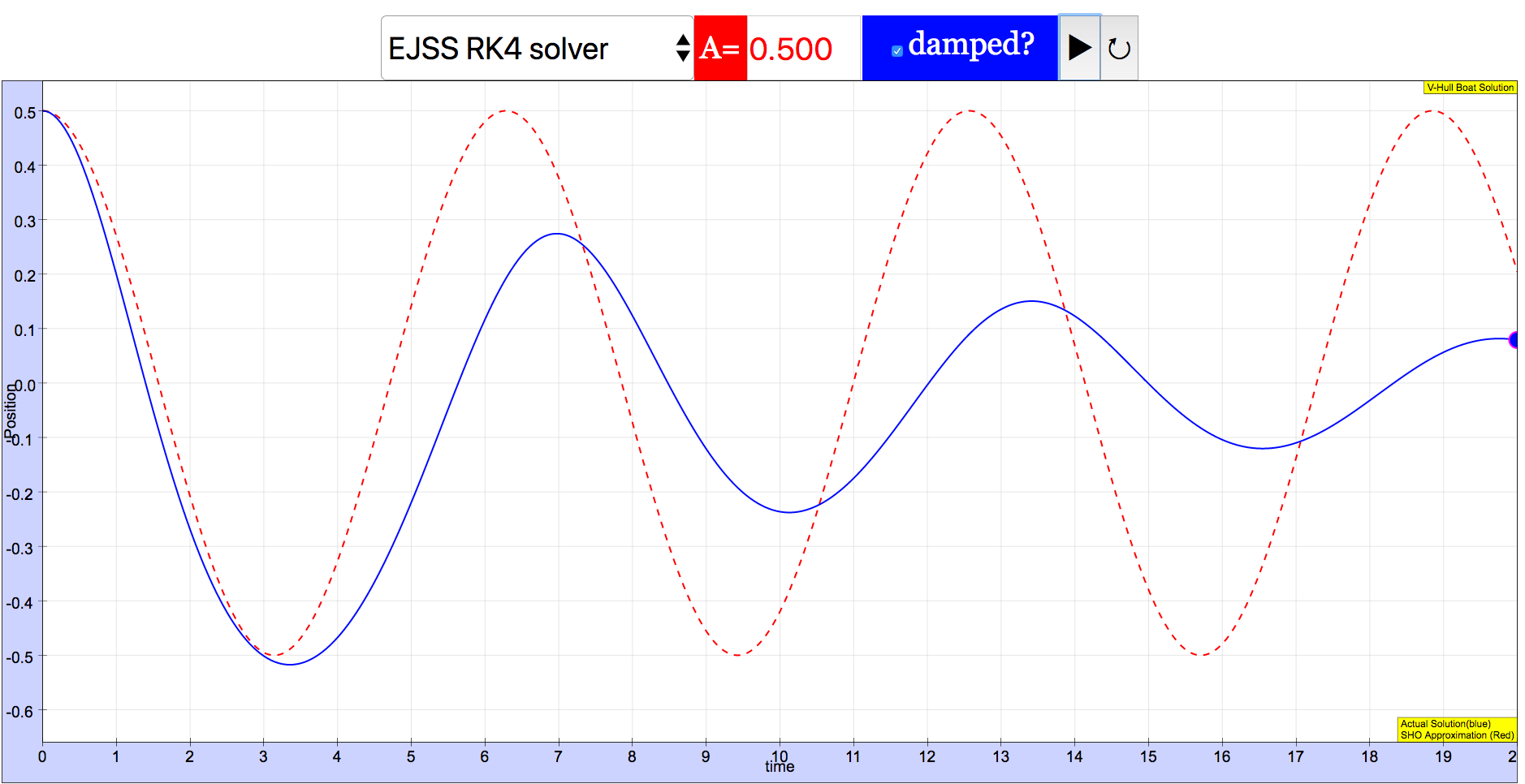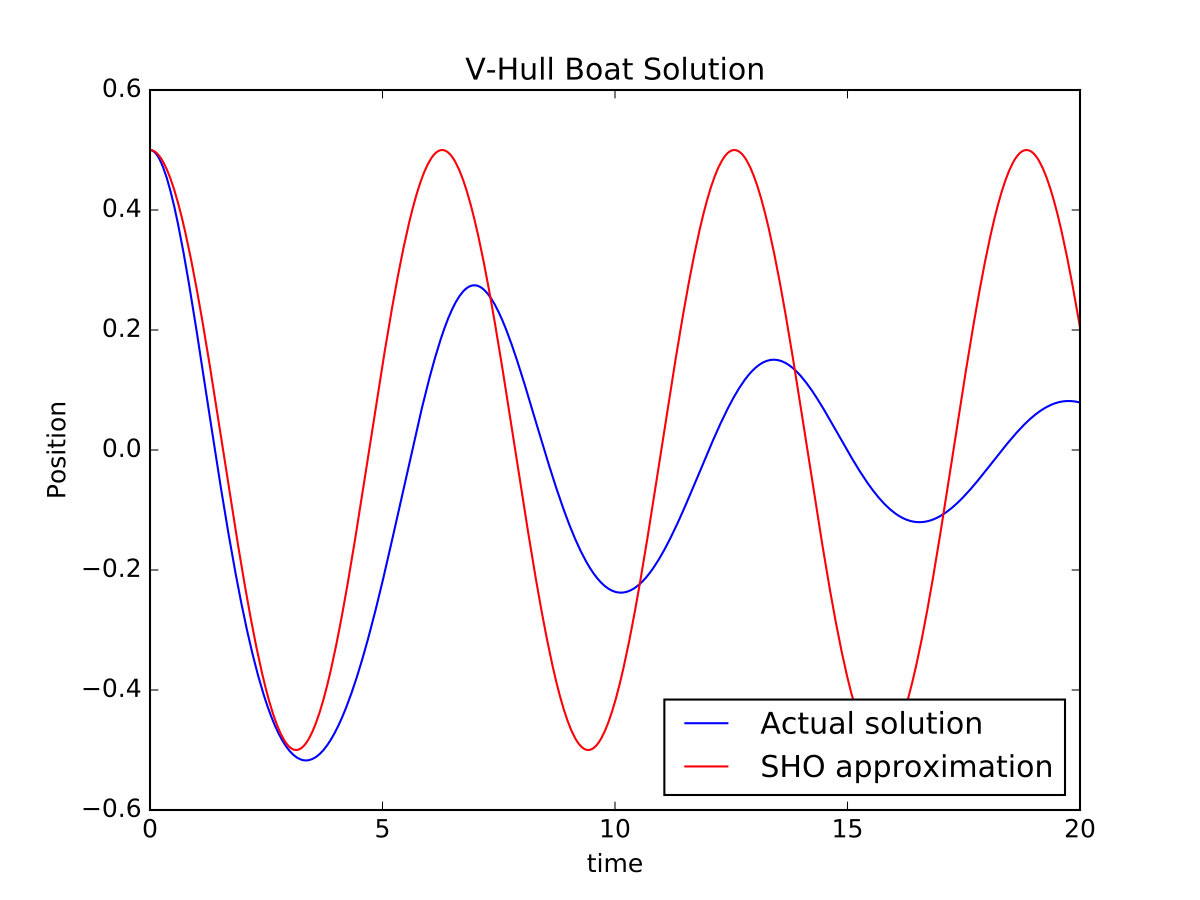### Translations

Code Language Translator Run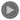### Software Requirements

SoftwareRequirements

 Android iOS Windows MacOS with best with Chrome Chrome Chrome Chrome support full-screen? Yes. Chrome/Opera No. Firefox/ Samsung Internet Not yet Yes Yes cannot work on some mobile browser that don't understand JavaScript such as..... cannot work on Internet Explorer 9 and below

### Credits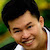Fremont Teng; Loo Kang Wee

[text]

### Instructions

#### Solver Combo Box

Toggle this combo box to select their respective solver.
Selecting the EJSS RK4 Solver includes a playable animation.

#### Amplitude Field Box

Adjust this to change the amplitude of the wave.

#### Damped Check Box

Toggling this will determine if the oscillation is damped or not.

(Not Damped)

(Damped)

#### Play/Pause and Reset Buttons

Plays/Pauses and resets the simulation respectively.

That is all, do enjoy the simulation in the link below:

Research

[text]

[text]

[text]

# Testimonials (0)

There are no testimonials available for viewing. Login to deploy the article and be the first to submit your review!